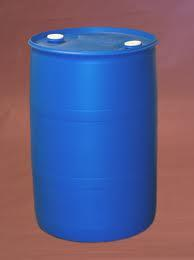# Buckets 2811

The barrel is filled with water up to 57% of its volume. When we add two buckets of water, we will fill it to 73% of its volume. What is the volume of the barrel if the volume of the bucket is 12 liters?

V =  150 l

### Step-by-step explanation:

0.57·V + 2·12 = 0.73·V

0.16V = 24

V = 24/0.16 = 150

V = 150

Our simple equation calculator calculates it.Did you find an error or inaccuracy? Feel free to write us. Thank you!

Tips for related online calculators